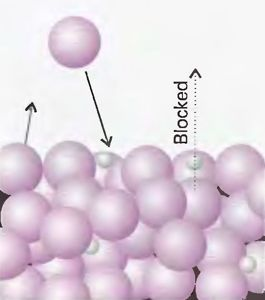Raoult's Law : Partial Vapour Pressure, Equation, Limitations

What is Raoult’s law?

According to Raoult’s Law, for a solution of volatile liquids, the partial vapour pressure of each component (volatile) of the solution is proportional to the mole fraction of that component present in the solution.

Understanding Raoult’s law

The study of colligative properties has been one of the most advanced subjects of research in physical chemistry. The properties of solutions such as partial pressure and vapour pressure and their subsequent variation due to varying parameters have been a pivotal point for the developments in this field. Raoult’s Law gives us a quantitative relation between the partial vapour pressure of components present in the vapour solution phase above a liquid solution and the mole fraction of that component in the solution. Raoult’s Law is defined as a solution that has one or more volatile liquids in it.Let us try to understand what exactly Francois Marie Raoult wanted to describe through his statement given as Raoult’s Law. As for now suppose we have a liquid binary solution consisting of a solvent 1 and a volatile solute 2. Note that this law is defined only for volatile components of a solution. Let Ptotal be the total vapour pressure of the two components and P1 and P2 be the partial vapour pressures of component 1 and component 2 respectively, and the mole fraction of component 1 and 2 be x1 and x2 in the solution phase and y1 and y2 in the vapour phase respectively.

Raoult’s law Equation

So, for component 1:

P1    α    x1

P1 = P1o x1,……………………………. (1)

Where P1o is the vapour pressure of component 1 in a pure state.

Similarly for component 2:

P2 = P2 o x2, …………………………….. (2)

From Dalton’s Law of partial pressures we know that,

Ptotal = P1+P2

Using the values of P1 and P2 from equation (1) and (2) respectively we have:

Ptotal = P1ox1 + P2ox2

=>  Ptotal = P1o(1-x2) + P2ox2

=>  Ptotal = P1o + (P2o-P1o)x2

We can draw the following inferences from the above equation:

1. The above equation is a straight line between Ptotal and x2 whose slope is given by (P2o-P1o) and the y-intercept is equal to P1o.
2. The total vapour pressure above a solution varies linearly with the mole fraction of component 2.
3. The total vapour pressure above a solution depends on the vapour pressure of Component 1 and 2 in their pure state and the mole fraction of component 2 in the solution.

Limitations of Raoult’s Law

The above-discussed topic finds application in many industries, but developments in science and technology have rendered even Raoult’s Law some limitations.

• It is applicable only to ideal liquid solutions.
• The results obtained for some complex, concentrated solutions that have significant intermolecular forces varying considerably from the law.

Nevertheless, the law applies to most ideal solutions and has been a base for further studies in this field.

To clarify further doubts, register with BYJU’S and download BYJU’S The Learning App or get in touch with our mentoring team.

Frequently Asked Questions on Raoult’s Law

What is Raoult’s law?

According to Raoult’s Law, for a solution of volatile liquids, the partial vapour pressure of each component (volatile) of the solution is proportional to the mole fraction of that component present in the solution.

What are the Limitations of Raoult’s Law ?

It is applicable only to ideal liquid solutions and The results obtained for some complex, concentrated solutions that have significant intermolecular forces varying considerably from the law.